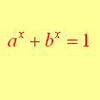#### You may also like### Growing

Which is larger: (a) 1.000001^{1000000} or 2? (b) 100^{300} or 300! (i.e.factorial 300)### What Do Functions Do for Tiny X?

Looking at small values of functions. Motivating the existence of the Taylor expansion.### Equation Attack

The equation a^x + b^x = 1 can be solved algebraically in special cases but in general it can only be solved by numerical methods.

# Building Approximations for Sin(x)

##### Age 16 to 18Challenge Level

As a brute force approach you can choose 4 values of x, work out sin(x) and then solve the 4 simultaneous equations in 4 variables. This will give a polynomial which matches the values for your choices of x.

However, you can save time by noting the symmetry properties of sin(x), in that sin(-x) = - sin(x).